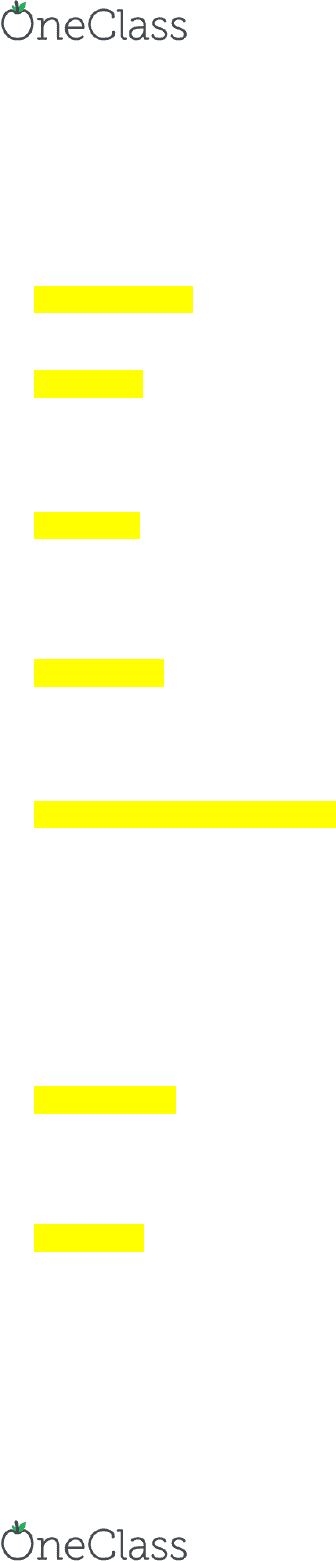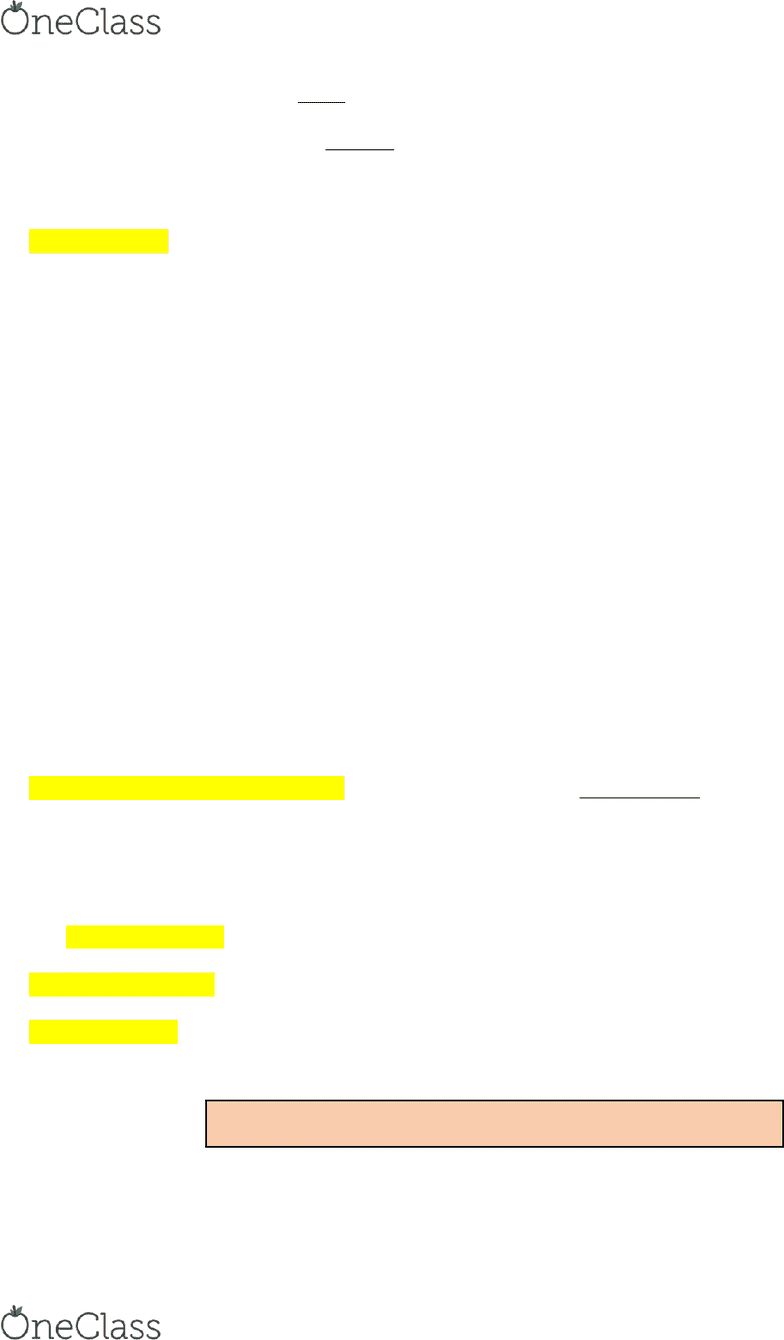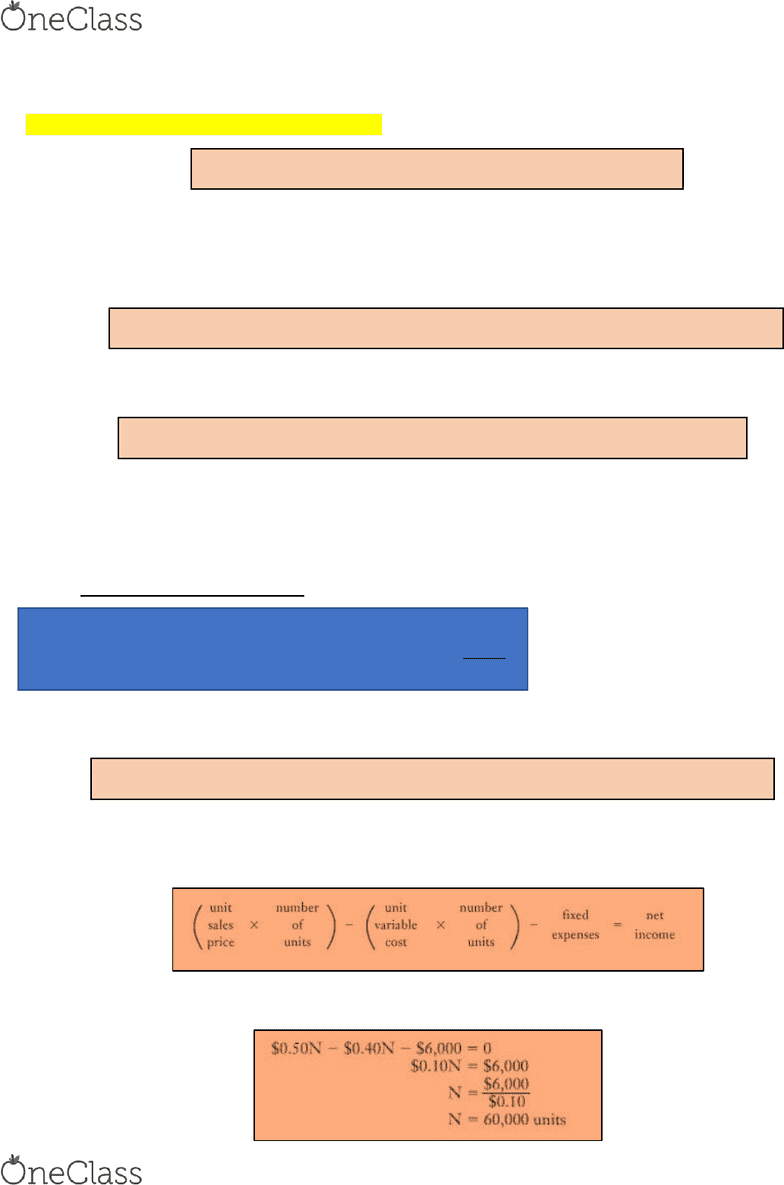Textbook Notes (290,000)
CA (170,000)
COMM (100)
Chapter 2

# COMM-2016EL Chapter Notes - Chapter 2: Unit, Longrun, Lincoln Near-Earth Asteroid Research

Department
Course Code
COMM-2016EL
Professor
Kayla Levesque
Chapter
2

This preview shows pages 1-2. to view the full 8 pages of the document.Chapter 2 Cost Behaviour ad Cost Volue
Relatioships
What will happen to financial results if a specified level of activity or volume fluctuates? Answering this
question is the first step in analyzing cost behaviour…
Cost Behaviour how the activities of an organization affect its costs
A knowledge of patterns & cost behaviour offers valuable insights in planning & controlling operations
Short-Run a period when productive capacity cannot be changed and must be accepted as a constraint
The short-run is usually considered to be one year or less, although can be longer in some industries
Calculations related to cost behaviour (CVP analysis) are most relevant in the short-run because large
and significant capacity constraints are fixed
Long-Run a period when productive capacity can be changed and need not be considered a constraint
Cost behaviour is still important in the long run because organizations can plan to alter constraints and
they must anticipate how this will affect cost behaviour
Cost Drivers
Cost Drivers activities that affect costs
An organization has many cost drivers across its value chain
How well the accountant does at identifying he most appropriate cost drivers determines how well
managers understand cost behaviour and how well costs are controlled
Volume-Related Cost Drivers include the number of orders processed, number of items billed in a billing
department, number of admissions to a theatre, number of kg handled in a warehouse, number of hours worked
in the assembly department, etc.
All of these cost drivers can serve either directly or indirectly as a measure of the volume of output of
goods or services
Comparison of Variable & Fixed Costs
Costs are classified as variable or fixed depending on how much they change as the level of a particular cost
driver changes…
Variable Cost a cost that changes in direct proportion to changes in the cost driver
(Example: Door-to-door salesman is paid 40% commission on their sales. Thus, sales commissions is a
variable cost with respect to sales revenues)
Fixed Cost a cost that is not immediately affected by changes in the cost driver
A fixed cost does not change IN TOTAL, but it becomes progressively smaller on a PER UNIT basis as
the volume increases
(Example: Sony rents a factory for \$500,000 per year… The total cost of rent is not affected by the number of
TV’s produced. However, the unit cost of rent applicable to each TV’s does depend on the total number of TV’s
produced)
find more resources at oneclass.com
find more resources at oneclass.com

Only pages 1-2 are available for preview. Some parts have been intentionally blurred.When predicting costs, 2 rules of thumb are useful:
1. Think of fixed costs as a total. Total fixed costs remain unchanged regardless of changes in cost-driver
activity
2. Think of variable costs on a per unit basis. The per unit variable cost remains unchanged regardless of
changes in cost-driver activity
RELEVANT RANGE
Relevant Range the limits of cost-driver activity within which a specific relationship between costs and the
cost driver is valid
Remember that even within the relevant range, a fixed cost remains fixed only over a given time period
usually the budget periodThese items are unlikely to change within a given year
A relevant range is usually listed in terms of volume of production, and if production falls below or rises
above the range, the fixed costs would change
The basic idea of relevant range also applies to variable costs. Outside a relevant range, some variable
costs may behave differently per unit of cost-driver activity
(Example: rent costs will rise if increased production requires a larger or additional building or if the
landlord decides to raise the rent; or vice versa)
DIFFICULTIES IN CLASSIFYING COSTS
The possibility of costs behaving in a non-linear way (not behaving in a straight line)
Costs may be simultaneously affected by more than one cost driver
Depends on the decision situation… More costs are fixed and fewer are variable when decisions involve
very short time spans and very small increases in activities
For now, we shall assume that any cost may be classified as EITHER variable or fixed, and that a given variable
cost is associated with ONLY ONE volume-related cost driver, and that relationship is LINEAR
Cost-Volume-Profit Analysis
Cost-Volume-Profit (CVP) Analysis the study of the effects of output volume on revenue (sales), expenses
(costs), and net income (net profit)
BREAK EVEN POINT CONTRIBUTION MARGIN & EQUATION TECHNIQUES
The most basic CVP analysis computes the monthly break-even point in number of units and in dollar sales
The Break-Even Point is the level of sales at which revenue = expenses, and net income is ZERO
Break-Even Analysis the study of cost-volume-profit relationships
Margin of Safety shows how far sales can far below the planned level before losses occur
One direct use of the break-even point is to assess possible risks
Margin of Safety = Planned Unit Sales Break-Even Unit Sales
The 2 basic techniques of determining the break-even point are the contribution-margin technique and the
equation technique
find more resources at oneclass.com
find more resources at oneclass.com

Unlock to view full version

Only pages 1-2 are available for preview. Some parts have been intentionally blurred.1. Contribution-Margin Technique
Every unit sold generates a contribution margin (marginal income)
Unit Contribution Margin (Marginal Income) the sales price minus all the variable expenses
Unit Contribution Margin = Sales Price Variable Expenses
The breakeven point is reached when enough units have been sold to generate a total contribution
margin equal to the total fixed costs!
Divide the amount of fixed costs by the unit contribution margin to determine the number of units that
need to be sold to break even
Break Even Volume in Units (#) = Total Fixed Costs / Unit Contribution Margin
Determine the sales revenue at the breakeven point by taking the breakeven number of units multiplied
by the unit sales price
Sales Revenue @ Break Even Point = Break Even # of Units * Unit Sales Price
Sometimes, the unit price and unit variable costs are not known… This situation is common at companies that
sell more than one product because no single price or variable cost applied to all products at many different
prices…
In these cases, you must work with total sales and total variable costs, and calculate variable costs as a
percentage of each sales dollar
EX. Sales Price 100%
Variable costs as a percentage of dollar sales 80%
Contribution-margin percentage 20%
Therefore, 20% of each sales dollar is available for the recovery of fixed expenses and the making of net
income… It is based on dollar sales without determining the break-even point in units
Break Even Volume in Dollars (\$) = Total Fixed Costs / Contribution-Margin Percentage
2. Equation Technique
The equation technique is the most general form of analysis the one that may be adapted to any conceivable
cost-volume-profit situation
At the break-even point, net income is 0… Let N be the # of units sold to break even. Therefore, an example
would be:
find more resources at oneclass.com
find more resources at oneclass.com